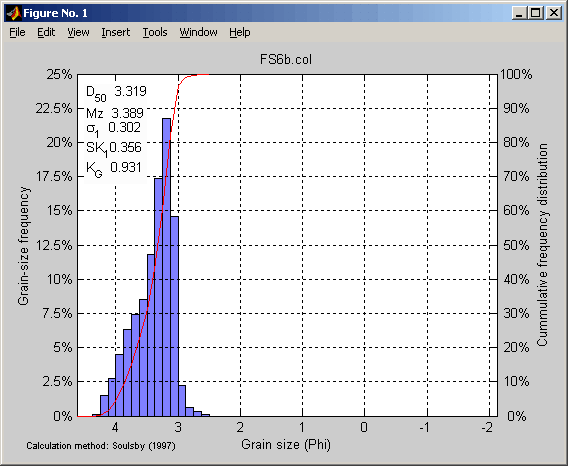cal_settling.m

Introduction
Cal_settling is a Matlab routine (that means Matlab must be installed in order to use cal_settling) that processes the data of a settling column for grain-size analysis. It converts the scale output of the settling column into a grain-size distribution, computes the usual grain-size statistics and produces also a graphic output.

Full help how to use it is included in the comments at the beginning of the routine.

cal_settling.m (version 1.17, 22 kB)

Detailed description
• Cal_settling was written to process the data of the settling column of the School of Ocean and Earth Science, Southampton Oceanographic Centre, University of Southampton.
• The input data must be the readings of the scale (units do not matter) recorded with a fixed time-step. The time-step MUST be written as a constant in the routine. The input data can either be given as a text file with one weight record per line (and nothing else), or as Matlab array.
• The routine can use 4 different formulae for converting the settling velocity into grain size: Gibbs et al. (1971), Baba and Komar, (1981), van Rijn (1993) and Soulsby (1997). The used formula is selected by a constant in the routine. It is recommended to use the formula of Soulsby (1997).
• The results are given in Phi units, by default with 1/8 Phi resolution, but other resolutions can be selected.
• It is possible to average together several analyses, for example replicates produced from sub-samples, by giving the multiples filenames in a cell array of strings. Different temperatures or settling heights can be specified. It is strongly recommended to FIRST process each replicate separately and to examine the quality and the similarity of the results BEFORE averaging the replicates of one sample.
• Cal_setting produces a figure representing the grain-size frequencies and the cumulative frequency distribution (see example below). The statistical parameter (either from the graphical method or the moment method, selection through a constant in the routine) can be printed on this figure. The figure drawing can be deactivated with an option. This figure can be printed from Matlab, or copied-pasted from Matlab into another program.
• With a particular option, the routine copy the numerical results in the system clipboard, so that they can be pasted into another program like Excel.
• The routine can also return the settling velocities for a given temperature and grain-size density.
• The settling column in Southampton often records a slight mass increase in the first seconds, which does not correspond to settling grains but represent some noise. This produces a peak in the coarsest grain-size class. To remove this effect, use the option "ixx", which ignore the xx first seconds (example: "i08" for 8 seconds).
• Explanation of the calling syntax is obtained by typing "help cal_settling" at the Matlab prompt. Additional help and explanations are in the firsts 70 comment lines of the routine.

Examples of usage

 cal_settling('FS6b.col',20,[],1.85) simple use with temperature 20°C, settling height 1.85 m cal_settling('FS6b.col',20,2800,1.85) with grain density 2800 kg m-3 cal_settling('FS6b.col',20,[],1.85,'nofig') without drawing a figure (useful inside loops) cal_settling('FS6b.col',20,[],1.85,'copy') numerical results copied into the system clipboard cal_settling('FS6b.col',20,[],1.85,1/4) with results at 1/4 Phi resolution cal_settling('FS6b.col',20,[],1.85,'i08') ignore the first 8 seconds cal_settling({'FS6a.col','FS6b.col'},20,[],1.85) average two analyses with the same temperature cal_settling({'FS6a.col','FS6b.col'},[20,21],[],1.85) average two analyses with different temperatures distribution = cal_settling(...) return grain-size distribution in two columns (finer limit of grain-size classes and sediment-mass proportion [distribution,classes] = cal_settling(...) return distribution (1 column with sediment-mass proportion) and separated variables with limits of grain-size classes) [distribution,classes,stat] = cal_settling(...) return also the grain-size statistics Ws=cal_settling([],20) return the settling velocities in m/s

Produced figureReferences

Gibbs R.J., Matthews M.D. and Link D.A. (1971) The relationship between sphere size and settling velocity. Journal of sedimentary Petrology, 41/1, 7-18.
Baba J. & Komar P.D. (1981) Measurements and analysis of settling velocities of natural quartz sand grains. Journal of sedimentary Petrolology, 51, 631-640.
van Rijn L.C. (1993) Principles of sediment transport in rivers, estuaries and coastal seas. Aqua Publications.
Soulsby R. (1997) Dynamics of marine sands: a manual for practical applications. Thomas Telford.

Back to the main page